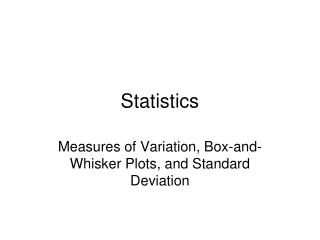Download PresentationStatistics

# Statistics - PowerPoint PPT PresentationDownload Presentation## Statistics

- - - - - - - - - - - - - - - - - - - - - - - - - - - E N D - - - - - - - - - - - - - - - - - - - - - - - - - - -
##### Presentation Transcript

1. Statistics Measures of Variation, Box-and-Whisker Plots, and Standard Deviation

2. range: difference between the greatest and least values in the set of data lower quartile: median of the lower half of the data upper quartile: median of the upper half of the data interquartile range: difference between the upper and lower quartiles outliers: any value that is at least 1.5 interquartile ranges beyond the upper or lower quartile boundaries: UQ + 1.5IQR (upper limit) LQ – 1.5IQR (lower limit)

3. Find the range, quartiles, interquartile range, and outliers for each data set. 1. 4,1,2,7,7,5,4,1,8,20,2,11,7,7,1

4. For a box-and-whisker plot, the following items must be found: quartiles, least value and greatest value that is not an outlier, and outliers Make a box-and-whisker plot for the following. 3. 4,1,2,7,7,5,4,1,8,20,2,11,7,7,1

5. Follow these steps: (explanation/breakdown of formula) • Find the mean. • Find the difference between each value and the mean. • Square these differences. • Find the mean of the squares. 5. Take the square root of the number from step 4.

6. Find the standard deviation for each set of data. 5. {11,7,2,4,1}

7. 6. {29,45,38,51,47,39,37,40,36,48}